Download Presentation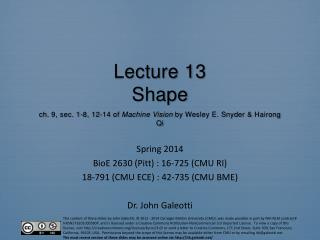Lecture 13 Shape ch . 9, sec. 1-8, 12-14 of Machine Vision by Wesley E. Snyder & Hairong Qi

# Lecture 13 Shape ch . 9, sec. 1-8, 12-14 of Machine Vision by Wesley E. Snyder & Hairong Qi - PowerPoint PPT Presentation

Lecture 13 Shape ch . 9, sec. 1-8, 12-14 of Machine Vision by Wesley E. Snyder & Hairong Qi. Spring 2014 BioE 2630 (Pitt) : 16-725 (CMU RI) 18 -791 (CMU ECE) : 42-735 (CMU BME) Dr. John Galeotti. Shape Analysis. Image analysis requires quantification of image contentsI am the owner, or an agent authorized to act on behalf of the owner, of the copyrighted work described.
Download Presentation## Lecture 13 Shape ch . 9, sec. 1-8, 12-14 of Machine Vision by Wesley E. Snyder & Hairong Qi

An Image/Link below is provided (as is) to download presentation

Download Policy: Content on the Website is provided to you AS IS for your information and personal use and may not be sold / licensed / shared on other websites without getting consent from its author.While downloading, if for some reason you are not able to download a presentation, the publisher may have deleted the file from their server.

- - - - - - - - - - - - - - - - - - - - - - - - - - E N D - - - - - - - - - - - - - - - - - - - - - - - - - -
Presentation Transcript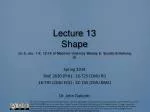### Lecture 13Shapech.9, sec. 1-8, 12-14 of Machine Vision by Wesley E. Snyder & Hairong Qi

Spring 2014

BioE 2630 (Pitt) : 16-725 (CMU RI)

18-791 (CMU ECE) : 42-735 (CMU BME)

Dr. John Galeotti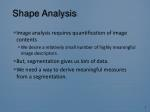Shape Analysis
• Image analysis requires quantification of image contents
• We desire a relatively small number of highly meaningful image descriptors.
• But, segmentation gives us lots of data.
• We need a way to derive meaningful measures from a segmentation.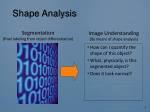Shape Analysis
• How can I quantify the shape of this object?
• What, physically, is this segmented object?
• Does it look normal?

Segmentation

(Pixel labeling from object differentiation)

Image Understanding

(By means of shape analysis)Shape Analysis &Linear Transformations
• We want to identify objects…
• Based on numerical shape descriptors.
• But:
• Changing the the zoom (size), position, or orientation of an object (or the “camera”) changes the contents of the resulting image.
• We often need…
• Shape descriptors that evaluate to the same (vector or scalar) value for all sizes, positions, and/or orientations of any given shape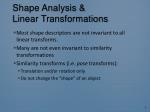Shape Analysis &Linear Transformations
• Most shape descriptors are not invariant to all linear transforms.
• Many are not even invariant to similarity transformations
• Similarity transforms (i.e. pose transforms):
• Translation and/or rotation only
• Do not change the “shape” of an object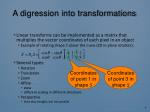A digression into transformations
• Linear transforms can be implemented as a matrix that multiplies the vector coordinates of each pixel in an object
• Example of rotating shape S about the z-axis (2D in-plane rotation):
• Several types:
• Rotation
• Translation
• Zoom
• Affine
• skew
• different scaling in different directions
• Perspective
• lines stay straight, but not parallel

Coordinates

of point 1 in

shape S

Coordinates

of point 3 in

shape S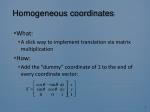Homogeneous coordinates
• What:
• A slick way to implement translation via matrix multiplication
• How:
• Add the “dummy” coordinate of 1 to the end of every coordinate vector:Transformations for Medical Imaging
• In medical imaging, we usually don’t have optical perspective.
• So, we usually don’t want or need invariance to perspective transformations.
• We often don’t even need affine transforms.
• In medical imaging, we know the size of each voxel.
• So, in some cases, we don’t want or need invariance to scale/zoom either.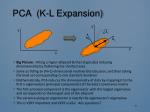PCA (K-L Expansion)

y’

y

b2

b1

x’

x

• Big Picture: Fitting a hyper-ellipsoid & then (typically) reducing dimensionality by flattening the shortest axes
• Same as fitting an (N+1)-dimensional multivariate Gaussian, and then taking the level set corresponding to one standard deviation
• Mathematically, PCA reduces the dimensionality of data by mapping it to the first n eigenvectors (principal components) of the data’s covariance matrix
• The first principal component is the eigenvector with the largest eigenvalue and corresponds to the longest axis of the ellipsoid
• The variance along an eigenvector is exactly the eigenvector’s eigenvalue
• This is VERY important and VERY useful. Any questions?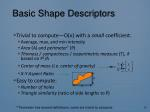Basic Shape Descriptors
• Trivial to compute—O(n) with a small coefficient:
• Average, max, and min intensity
• Area (A) and perimeter* (P)
• Thinness / compactness / isoperimetric measure (T), if based on P2/A
• Center of mass (i.e. center of gravity)
• X-Y Aspect Ratio
• Easy to compute:
• Number of holes
• Triangle similarity (ratio of side lengths to P)

y

x

* Perimeter has several definitions; some are trivial to computeBasic Shape Descriptors

y

• Requires PCA first, which itself is O(D3+D2n):
• Approximate minimum aspect ratio
• Approximate diameter (D)
• Thinness / compactness / isoperimetric measure (T), if based on D/A
• O(n log n):
• Convex discrepancy
• Difficult to compute:
• Exact diameter = absolute max chord
• Exact minimum aspect ratio
• Symmetry, mirror or rotational

x

* Perimeter has several definitions; some are difficult to computeMethod of Normalization
• Idea: Transform each shape’s image region into a canonical frame before attempting to identify shapes
• Simple, but common, example:
• Move origin to the center of gravity (CG) of the current shape
• Used by central moments (next slide)
• Complex example:
• Attempt to compute and apply an affine transform to each object such that all right-angle-triangle objects appear identicalMoments
• Easy to calculate
• Sequence of derivation:
• Moments: mpq = ∑ xpyqf(x,y)
• Central moments: pq (origin @ CG)
• Normalized central moments: pq
• Invariant to translation & scale
• Invariant moments: n
• Invariant to translation, rotation, & scale
• Only 7 of them in 2D
• Equations are in the text
• Problem: Sensitive to quantization & samplingChain codes

3

4

2

0

5

1

=312318781

6

8

7

• Describe the boundary as a sequence of steps
• Typically in 2D each step direction is coded with a number
• Conventionally, traverse the boundary in the counter-clockwise direction
• Useful for many things, including syntactic pattern recognitionFourier Descriptors
• Traverse the boundary
• Like for chain codes
• But, take the FT of the sequence of boundary-point coordinates
• In 2D, use regular FT with i = y-axis
• Equivalences make invariance “easy”:
• Translation = DC term
• Scale = multiplication by a constant
• Rotation about origin = phase shift
• Problem: Quantization errorMedial Axis
• I may revisit this in another lecture (if time allows)
• For now:
• Locus of the centers of the maximal bi-tangent circles/spheres/…Deformable Templates
• Represent a shape by the active contour that segments it
• Deforming the contour deforms the shape
• Two shapes are considered similar if the boundary of one can be “easily” deformed into the boundary of the other.
• E.g., “easy” = small strain on the deformed curve and low energy required to deform the curveGeneralized Cylinders (GCs)
• Fit a GC to a shape
• This can be challenging
• Get two descriptive functions:
• Axis of the GC
• A vector-valued function
• Radius along the axis
• Typically a scalar-valued function